## LetsPlayMaths.Com

WELCOME TO THE WORLD OF MATHEMATICS

# Class 4 LCM and HCF

Lowest Common Multiples (LCM)

Highest Common Factor (HCF)

LCM / HCF Test

LCM/HCF Worksheet

## Lowest Common Multiples (LCM)

The lowest common multiple of two or more numbers is the smallest number that is a multiple of both the numbers. There are different methods by which we can find out the LCM of different numbers.

Listing Method

First list out the multiples of given numbers and then find out the smallest common multiple.

Please have a look at the below given video for better understanding.

Let's have a look at some examples given below.

Example 1. Find out the LCM of 2 and 3?

Solution.

Multiples of 2 = 2, 4, 6, 8, 10, 12, 14, 16, 18, 20

Multiples of 3 = 3, 6, 9, 12, 15, 18, 21, 24, 27, 30

Common multiples of 2 & 3 are 6, 12, 18

So, the lowest multiple of 2 & 3 is 6.

Example 2. Find out the LCM of 3 & 8?

Solution.

Multiples of 3 = 3, 6, 9, 12, 15, 18, 21, 24

Multiples of 8 = 8, 16, 24, 32, 40

Common multiples of 3 and 8 is 24.

So, the lowest multiple of 3 and 8 is 24.

When two numbers have no common factors other than 1, then their Lowest Common Multiple is the product of these two numbers.

Example 1. Find out the LCM of 3 and 7.

Solution. LCM of 3 and 7 = 3 × 7 = 21.

Example 2. Find out the LCM of 5 and 9.

Solution. LCM of 5 and 9 = 5 × 9 = 45

Prime Factor Method

In this method, we take the numbers and divide them with the smallest possible prime factor. If any number gets divided fully then we divide it, else we keep it as it is. In the next step, we divide it by the next possible prime factor. We keep on doing it unless we get all the quotients become 1. In the end, we multiply all the prime factors to get the LCM.

Please have a look at the below given video for better understanding.

Let's have a look at some example given below.

Example 1. Find out the LCM of 4 and 8.

Solution.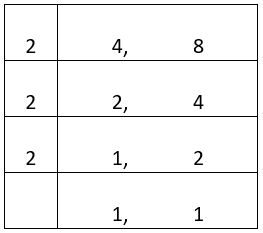Step 1. Divide 4 and 8 by 2 and write the quotient below

Step 2. Divide 2 and 4 by 2 and write the quotient below

Step 3. From 1 and 2, 1 can not be divided by 2, so leave it. Divide 2 by 2, quotient is 1, write both 1s below

Step 4. Now multiply all the prime factors. 2 × 2 × 2 = 8

So, the LCM of 4 and 8 is 8.

Example 2. Find LCM of 4, 6, and 8.

Solution.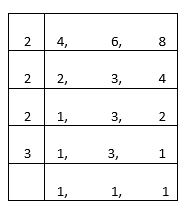LCM = 2 × 2 × 2 × 3 = 24

Example 3. Find the LCM of 10, 15 and 25.

Solution.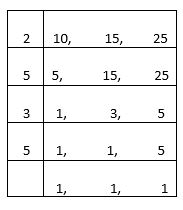LCM = 2 × 5 × 3 × 5 = 150

## Highest Common Factor (HCF)

HCF of two numbers is the highest factor which is common to the given numbers. That means the greatest number which divides the given numbers fully. We have various method to determine HCF of given numbers.

Listing Method

In this method we have to list down all the factors of the given numbers, and then we have to determine the highest common factor out of them.

Please have a look at the below given video for better understanding.

Let's have a look at some examples.

Example 1. Find out the HCF of 8 and 12.

Solution.

Factors of 8 = 1, 2, 4, 8

Factors of 12 = 1, 2, 4, 6, 12

Common factors are 1, 2, and 4, among these common factors 4 is the highest factor.

So, 4 is the HCF of 8 and 12.

Example 2. Find out the HCF of 24 and 36.

Solution.

Factors of 24 = 1, 2, 3, 4, 6, 8, 12, 24

Factors of 36 = 1, 2, 3, 4, 6, 9, 12, 18, 36

Common factors are 1, 2, 3, 4, 6, and 12.

So, 12 is the HCF of 24 and 36.

Prime Factor Method

We need to factorize each number as shown below and multiply all the common prime factors to get the HCF.

Please have a look at the below given video for better understanding.

Let's have a look at some examples.

Example 1. Find out the HCF of 8 and 12.

Solution.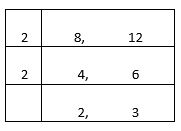Factorize both the numbers until they have common Factors. So, we have two common prime factors.

2 X 2 = 4

So, the HCF of 8 and 12 is 4.

Example 2. Find out the HCF of 8, 24 and 32?

Solution.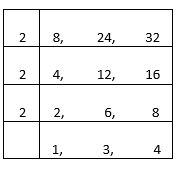1. Here 2 is the common prime factor of 8, 24 and 32.

2. Here 2 is the common prime factor of 4, 12 and 16.

3. Here 2 is the common prime factor of 2, 6 and 8.

So, we have three common prime factors.

2 X 2 X 2 = 8

HCF of 8, 24 and 32 is 8.

## LCM / HCF Test

LCM & HCF Test - 1

## Class-4 LCM and HCF Worksheet

LCM & HCF Worksheet - 1

LCM & HCF Worksheet - 2

LCM & HCF Worksheet - 3